# NCERT Solutions: Algebraic Expressions- 3 Notes | Study Mathematics (Maths) Class 7 - Class 7

## Class 7: NCERT Solutions: Algebraic Expressions- 3 Notes | Study Mathematics (Maths) Class 7 - Class 7

The document NCERT Solutions: Algebraic Expressions- 3 Notes | Study Mathematics (Maths) Class 7 - Class 7 is a part of the Class 7 Course Mathematics (Maths) Class 7.
All you need of Class 7 at this link: Class 7

Q.1. Observe the patterns of digits made from line segments of equal length. You will find such segmented digits on the display of electronic watches or calculators.
a)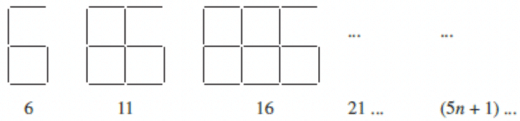b)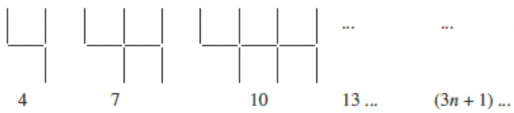c)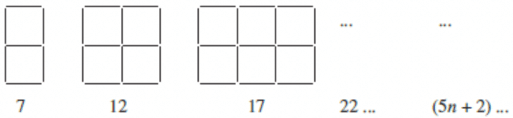If the number of digits formed is taken to be n, the number of segments required to form n digits is given by the algebraic expression appearing on the right of each pattern. How many segments are required to form 5, 10, 100 digits of the kind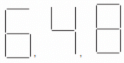Solution.

(a) From the question it is given that the numbers of segments required to form n digits of the kind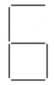is (5n + 1)
Then, the number of segments required to form 5 digits = ((5 × 5) + 1)
= (25 + 1)
= 26
The number of segments required to form 10 digits = ((5 × 10) + 1)
= (50 + 1)
= 51
The number of segments required to form 100 digits = ((5 × 100) + 1)
= (500 + 1)
= 501
(b) From the question it is given that the numbers of segments required to form n digits of the kind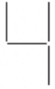is (3n + 1)
Then, the number of segments required to form 5 digits = ((3 × 5) + 1)
= (15 + 1)
= 16
The number of segments required to form 10 digits = ((3 × 10) + 1)
= (30 + 1)
= 31
The number of segments required to form 100 digits = ((3 × 100) + 1)
= (300 + 1)
= 301
(c) From the question, it is given that the numbers of segments required to form n digits of the kind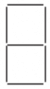is (5n + 2)
Then, the number of segments required to form 5 digits = ((5 × 5) + 2)
= (25 + 2)
= 27
The number of segments required to form 10 digits = ((5 × 10) + 2)
= (50 + 2)
= 52
The number of segments required to form 100 digits = ((5 × 100) + 1)
= (500 + 2)
= 502

Q.2. Use the given algebraic expression to complete the table of number patterns.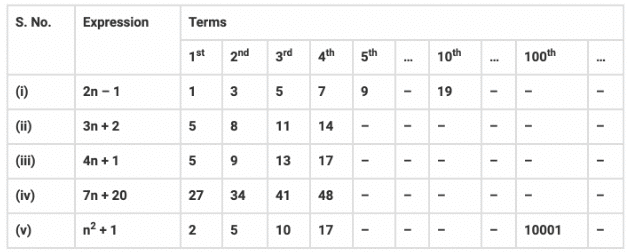Solution.
(i) From the table (2n – 1)
Then, 100th term =?
Where n = 100
= (2 × 100) – 1
= 200 – 1
= 199
(ii) From the table (3n + 2)
5th term =?
Where n = 5
= (3 × 5) + 2
= 15 + 2
= 17
Then, 10th term = ?
Where n = 10
= (3 × 10) + 2
= 30 + 2
= 32
Then, 100th term =?
Where n = 100
= (3 × 100) + 2
= 300 + 2
= 302
(iii) From the table (4n + 1)
5th term = ?
Where n = 5
= (4 × 5) + 1
= 20 + 1
= 21
Then, 10th term = ?
Where n = 10
= (4 × 10) + 1
= 40 + 1
= 41
Then, 100th term = ?
Where n = 100
= (4 × 100) + 1
= 400 + 1
= 401
(iv) From the table (7n + 20)
5th term =  ?
Where n = 5
= (7 × 5) + 20
= 35 + 20
= 55
Then, 10th term = ?
Where n = 10
= (7 × 10) + 20
= 70 + 20
= 90
Then, 100th term = ?
Where n = 100
= (7 × 100) + 20
= 700 + 20
= 720
(v) From the table (n2 + 1)
5th term = ?
Where n = 5
= (52) + 1
= 25+ 1
= 26
Then, 10th term = ?
Where n = 10
= (102) + 1
= 100 + 1
= 101
So the table is completed below.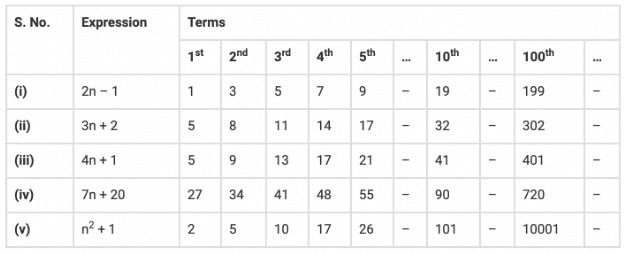The document NCERT Solutions: Algebraic Expressions- 3 Notes | Study Mathematics (Maths) Class 7 - Class 7 is a part of the Class 7 Course Mathematics (Maths) Class 7.
All you need of Class 7 at this link: Class 7Use Code STAYHOME200 and get INR 200 additional OFF

## Mathematics (Maths) Class 7

164 videos|237 docs|45 tests

Track your progress, build streaks, highlight & save important lessons and more!

,

,

,

,

,

,

,

,

,

,

,

,

,

,

,

,

,

,

,

,

,

;